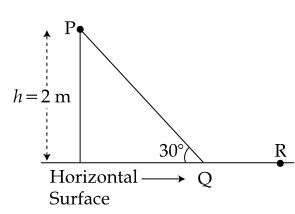#### A point particle of mass m, moves along the uniformly rough track PQR as shown in the figure.  The coefficient of friction, between the particle and the rough track equals µ.  The particle is released, from rest, from the point P and it comes to rest at a point R.  The energies, lost by the ball, over the parts, PQ and QR, of the track, are equal to each other, and no energy is lost when particle changes direction from PQ to QR. The values of the coefficient of friction µ and the distance x(=QR), are, respectively close to :Option: 1  0.2 and 6.5 m   Option: 3  0.2 and 3.5 m   Option: 4   0.29 and 6.5 mWork done by friction at QR = μmgx

In triangle, sin 30° = 1/2 = 2/PQ

PQ = 4 m

Work done by friction at PQ = μmg × cos 30° × 4 = μmg × √3/2 × 4 = 2√3μmg

Since work done by friction on parts PQ and QR are equal,

μmgx = 2√3μmg

x = 2√3 ≅ 3.5 m

Applying work energy theorem from P to R

decrease in P.E.=P.E.= loss of energy due to friction in PQPQ and QR

where h=2(given)